TitleCollege Algebra
Tutorial 5:  Rational ExponentsLearning Objectives

 After completing this tutorial, you should be able to: Rewrite a rational exponent in radical notation. Simplify an expression that contains a rational exponent. Use rational exponents to simplify a radical expression.Introduction

 In this tutorial we are going to combine two ideas that have been discussed in earlier tutorials: exponents and radicals.  We will look at how to rewrite, simplify and evaluate these expressions that contain rational exponents.   What it boils down to is if you have a denominator in your exponent, it is your index or root number.  So, if you need to, review radicals covered in Tutorial 4: Radicals.  Also, since we are working with fractional exponents and they follow the exact same rules as integer exponents, you will need to be familiar with adding, subtracting, and multiplying them. If you feel that you need a review, click on review of fractions.  To review exponents, you can go to Tutorial 2: Integer Exponents.  Let's move onto rational exponents and roots.Tutorial

 Rational Exponents and Roots If x is positive, p and q are integers and q is positive,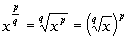In other words, when you have a rational exponent, the denominator of that exponent is your index or root number and the numerator of the exponent is the exponential part. I have found it easier to think of it in two parts.  Find the root part first and then take it to the exponential part if possible.  It makes the numbers a lot easier to work with.   Radical exponents follow the exact same exponent rules as discussed in Tutorial 2: Integer Exponents. In that tutorial we only dealt with integers, but you can extend those rules to rational exponents.  Here is a quick review of those exponential rules:

 Review of Exponential Rules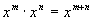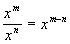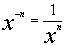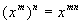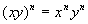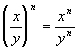Example 1: Evaluate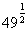.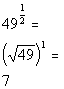*Rewrite exponent 1/2 as a square root

 We are looking for the square root of 49 raised to the 1 power, which is the same as just saying the square root of 49.  If your exponent's numerator is 1, you are basically just looking for the root (the denominator's exponent).  Our answer is 7 since the square root of 49 is 7.Example 2: Evaluate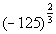.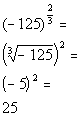*Cube root of -125 = -5

 In this problem we are looking for the cube root of -125 squared.  Again, I think it is easier to do the root part first if possible.  The numbers will be easier to work with.  The cube root of -125 is -5 and (-5) squared is 25.Example 3: Evaluate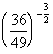.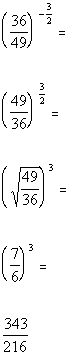*Rewrite as recip. of base raised to pos. exp. *DO NOT take the reciprocal of the exponent, only the base   *Square root of 49/36 = 7/6

 In this problem we have a negative exponent to start with.  That means we need to take the reciprocal of the base. Note that we DO NOT take the reciprocal of the exponent, only the base. From there we are looking for the square root of 49/36 cubed.  Again, I think it is easier to do the root part first if possible.  The numbers will be easier to work with.  The square root of 49/36 is 7/6 and 7/6 cubed is 343/216.Example 4: Simplify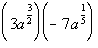.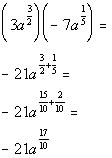Example 5: Simplify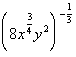.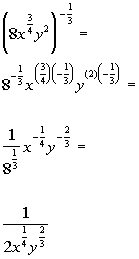*Cube root of 8 = 2Example 6: Simplify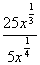.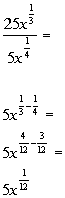Example 7:  Simplify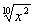by reducing the index of the radical. x represents positive real numbers.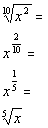*Simplify exponent *Rewrite exponent 1/5 as a fifth rootPractice Problems

 These are practice problems to help bring you to the next level.  It will allow you to check and see if you have an understanding of these types of problems. Math works just like anything else, if you want to get good at it, then you need to practice it.  Even the best athletes and musicians had help along the way and lots of practice, practice, practice, to get good at their sport or instrument.  In fact there is no such thing as too much practice. To get the most out of these, you should work the problem out on your own and then check your answer by clicking on the link for the answer/discussion for that  problem.  At the link you will find the answer as well as any steps that went into finding that answer.Practice Problems 1a - 1b: Evaluate the expression.

 1a.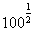(answer/discussion to 1a) 1b.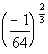(answer/discussion to 1b)Practice Problems 2a - 2c: Simplify the expression.

 2a.(answer/discussion to 2a) 2b.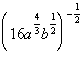(answer/discussion to 2b)

 2c.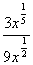(answer/discussion to 2c)Practice Problem 3a: Simplify the expression by reducing the index of the radical. x represents positive real numbers.

 3a.(answer/discussion to 3a)Need Extra Help on these Topics?

The following are webpages that can assist you in the topics that were covered on this page:

 http://www.wtamu.edu/academic/anns/mps/math/mathlab/int_algebra/int_alg_tut38_ratexp.htm This webpage helps you with rational exponents. http://www.purplemath.com/modules/exponent5.htm This webpage assists you with rational exponents.

Go to Get Help Outside the Classroom found in Tutorial 1: How to Succeed in a Math Class for some more suggestions.

Videos at this site were created and produced by Kim Seward and Virginia Williams Trice.
Last revised on Dec. 6, 2009 by Kim Seward.